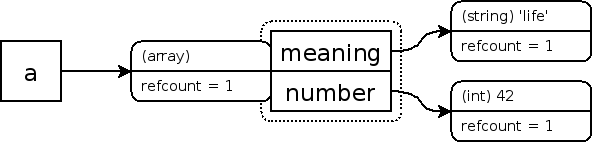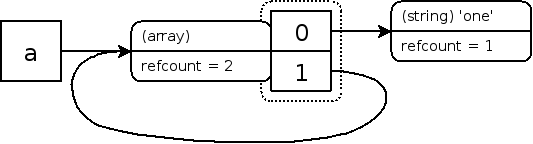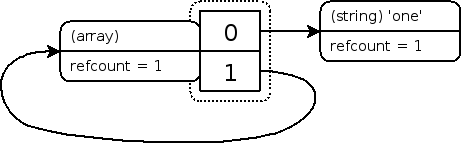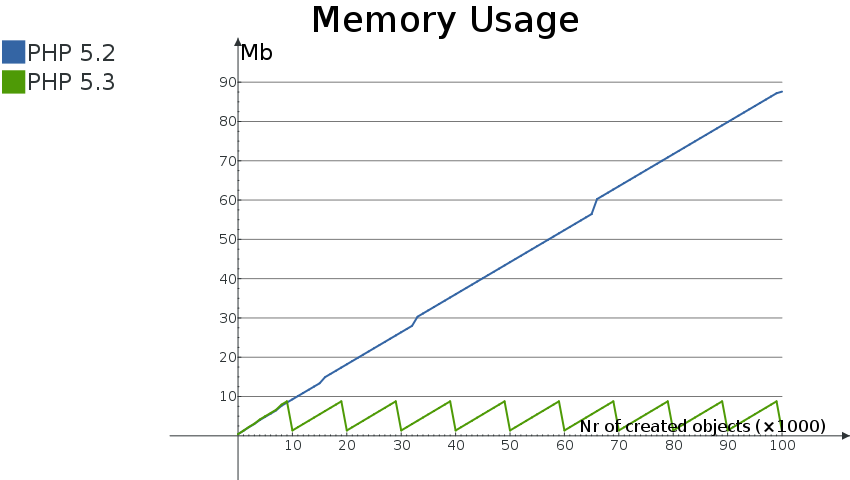#PHP的垃圾回收机制详解

0
0
01. 云栖社区>
2. 博客>
3. 正文

## PHP的垃圾回收机制详解

php中变量存在于一个zval的变量容器中。结构如下：

 类型 值 is_ref refcount

zval中，除了存储变量的类型和值之外，还有is_ref字段和refcount字段。

• is_ref：是个bool值，用来区分变量是否属于引用集合。什么意思呢，你可以这么认为：表示变量是否有一个以上的别名。
• refcount：计数器，表示指向这个zval变量容器的变量个数。

&引用赋值时，原变量的is_ref 变为1，refcount 加1.  如果给一个变量&赋值，之前 = 赋值的变量会分配空间。

```<?php
\$a = 1;
xdebug_debug_zval('a');
echo PHP_EOL;
\$b = \$a;
xdebug_debug_zval('a');
echo PHP_EOL;

\$c = &\$a;
xdebug_debug_zval('a');
echo PHP_EOL;

xdebug_debug_zval('b');
echo PHP_EOL;
?>
```

运行结果如下：

a:(refcount=1, is_ref=0),int 1

a:(refcount=2, is_ref=0),int 1

a:(refcount=2, is_ref=1),int 1

b:(refcount=1, is_ref=0),int 1

```<?php
\$a = array( 'meaning' => 'life', 'number' => 42 );
xdebug_debug_zval( 'a' );
echo PHP_EOL;
class Test{
public \$a = 1;
public \$b = 2;

function handle(){
echo 'hehe';
}
}

\$test = new Test();
xdebug_debug_zval('test');
?>
```

运行结果如下：

a:(refcount=1, is_ref=0),

```array
'meaning' => (refcount=1, is_ref=0),```

string

`'life' (length=4)`
`  'number' => (refcount=1, is_ref=0),`

int

` 42`

test:(refcount=1, is_ref=0),

```object(Test)
public 'a' => (refcount=2, is_ref=0),```

int

` 1`
`  public 'b' => (refcount=2, is_ref=0),`

int

`2`
` ````<?php
\$a = array( 'one' );
\$a[] =& \$a;
xdebug_debug_zval( 'a' );
?>
```

运行结果：

a:(refcount=2, is_ref=1),

```array
0 => (refcount=1, is_ref=0),```

string

` 'one' (length=3)`
`  1 => (refcount=2, is_ref=1), &array`

a 和 1 的zval容器 是一样的。如下：PHP5.3中，采用了专门的算法（比较复杂）。，来处理环状引用导致内存泄露的问题。

1：如果一个zval的refcount增加，那么此zval还在使用，肯定不是垃圾，不会进入缓冲区

2：如果一个zval的refcount减少到0， 那么zval会被立即释放掉，不属于GC要处理的垃圾对象，不会进入缓冲区。

3：如果一个zval的refcount减少之后大于0，那么此zval还不能被释放，此zval可能成为一个垃圾，将其放入缓冲区。PHP5.3中的GC针对的就是这种zval进行的处理。

```<?php
class Foo
{
public \$var = '3.1415962654';
}

\$baseMemory = memory_get_usage();

for ( \$i = 0; \$i <= 100000; \$i++ )
{
\$a = new Foo;
\$a->self = \$a;
if ( \$i % 500 === 0 )
{
echo sprintf( '%8d: ', \$i ), memory_get_usage() - \$baseMemory, "\n";
}
}
?>
``````<?php
class Foo
{
public \$var = '3.1415962654';
}

for ( \$i = 0; \$i <= 1000000; \$i++ )
{
\$a = new Foo;
\$a->self = \$a;
}

echo memory_get_peak_usage(), "\n";
?>
```

```
```

+ 关注